Download Presentation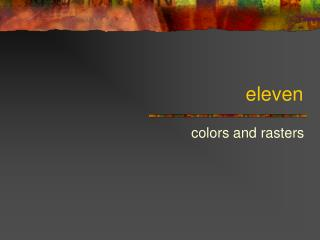eleven

# eleven - PowerPoint PPT Presentation

eleven. colors and rasters. Color objects. [color name ] Returns color with the specified name [color red green blue ] Returns color with the specified amounts of red, green, and blue light Arguments should be 0-255 [red c ] or c .R [green c ] or c .G [blue c ] or c .BI am the owner, or an agent authorized to act on behalf of the owner, of the copyrighted work described.
Download Presentation## eleven

An Image/Link below is provided (as is) to download presentation

Download Policy: Content on the Website is provided to you AS IS for your information and personal use and may not be sold / licensed / shared on other websites without getting consent from its author.While downloading, if for some reason you are not able to download a presentation, the publisher may have deleted the file from their server.

- - - - - - - - - - - - - - - - - - - - - - - - - - E N D - - - - - - - - - - - - - - - - - - - - - - - - - -
Presentation Transcript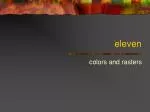### eleven

colors and rasters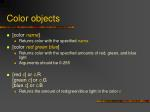Color objects
• [color name]
• Returns color with the specified name
• [color red green blue]
• Returns color with the specified amounts of red, green, and blue light
• Arguments should be 0-255
• [red c] or c.R[green c] or c.G[blue c] or c.B
• Returns the amount of red/green/blue light in the color c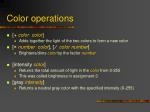Color operations
• [+ color color]
• Adds together the light of the two colors to form a new color
• [× number color], [ ⁄ color number]
• Brightens/dims color by the factor number
• [intensity color]
• Returns the total amount of light in the color from 0-255
• This was called brightness in assignment 3
• [gray intensity]
• Returns a neutral gray color with the specified intensity (0-255)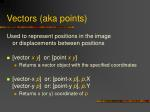Vectors (aka points)

Used to represent positions in the imageor displacements between positions

• [vector x y] or: [point x y]
• Returns a vector object with the specified coordinates
• [vector-x p] or: [point-x p],p.X[vector-y p] or: [point-yp],p.Y
• Returns x (or y) coordinate of p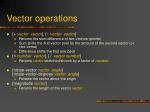Vector operations
• [+ vector vector], [− vector vector]
• Returns the sum/difference of two vectors (points)
• Sum shifts the first vector over by the amount of the second vector (or vice-versa)
• Difference shifts the first one back
• [× number vector], [ ⁄ vector number]
• Returns vector stretched/shrunk by a factor of number
• [rotate-vector vector angle][rotate-vector-degrees vector angle]
• Rotates the vector about the origin
• [magnitude vector]
• Returns the length of the vector vector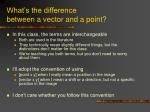What’s the differencebetween a vector and a point?
• In this class, the terms are interchangeable
• Both are used in the literature
• They technically mean slightly different things, but the distinctions don’t matter for this class
• We’re teaching you both terms, but you don’t need to worry about them
• I’ll adopt the convention of using
• [point x y] when I really mean a position in the image
• [vector x y] when I mean a shift or a direction, but not a specific position in the image
• I don’t care whether you follow this convention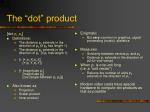[dot p1 p2]

Definitions:

The distance p1 extends in the direction of p2 (if p2 has length 1)

The distance p2 extends in the direction of p1 (if p1 has length 1)

[+ [× p1.X p2.X] [× p1.Y p2.Y]]

[× [magnitude p1] [magnitude p2] [cos [angle-between p1p2]]]

Also known as

Projection

Scalar product

Inner product

Enigmatic

But very common in graphics, signal processing (music), statistics

Measures

Similarity between vectors p1 and p2

Distance p1 extends in the direction of p2 (or vice-versa)

When p1 is one unit long(i.e. a “unit vector”)

Returns the number of units p2 extends in the direction of p1.

Modern video cards have special hardware to compute dot products as fast as possible

The “dot” product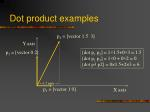Dot product examples

p2 = [vector 1.5 3]

Y axis

p3 = [vector 0 2]

[dot p1 p2] = 1×1.5+0×3 = 1.5

[dot p1 p3] = 1×0 + 0×2 = 0[dot p3 p2] = 0×1.5+2×3 = 6

←1.5 units →

p1 = [vector 1 0]

X axis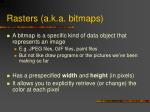Rasters (a.k.a. bitmaps)
• A bitmap is a specific kind of data object that represents an image
• E.g. JPEG files, GIF files, paint files
• But not like draw programs or the pictures we’ve been making so far
• Has a prespecified width and height (in pixels)
• It allows you to explicitly retrieve (or change) the color at each pixel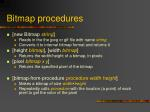Bitmap procedures
• [new Bitmap string]
• Reads in the the jpeg or gif file with name string
• Converts it to internal bitmap format and returns it
• [height bitmap], [width bitmap]
• Returns the width/height of a bitmap, in pixels
• [pixel bitmap x y]
• Returns the specified pixel of the bitmap
• [bitmap-from-procedure procedure width height]
• Makes a bitmap of size width×height
• Repeatedly calls procedure with the location (a point) of each pixel to get the color of the pixelExample

► [bitmap-from-procedure

[p → [color p.X p.X p.X]]

256

256]

The procedure[p → [color p.X p.X p.X]]

• Returns a color with equal amounts of red, green, and blue light
• So it’s grey
• It’s brightness varies with its horizontal positionComparing to iterated-group

► [bitmap-from-procedure

[p → [color p.X p.X p.X]]

256

256]

• This is sort of like iterated-group
• You give it a procedure
• And a number of times to run it(well, two numbers)
• And it makes a pictureComparing to iterated-group

► [bitmap-from-procedure

[p → [color p.X p.X p.X]]

256

256]

Except:

• The procedure you pass in
• Returns a color, not a shape
• Takes a position as an input
• Takes two count parameters (one for width, one for height)Example 2

► [bitmap-from-procedure

[p → [color p.X 0 p.Y]]

256

256]

The procedure[p → [color p.X 0 p.Y]]

• Changes red and blue independently
• So hue varies over spaceExample 3

► [define sine-wave

[n → [+ 128

[× 127 [sin [∕ n 20]]]]]]

<Procedure sine-wave>

► [bitmap-from-procedure [p → [color 0

[sine-wave p.X]

[sine-wave p.Y]]]

256

256]Bitmap procedures
• [map-bitmap procedure bitmap]
• Makes a new bitmap of the same size as bitmap
• Calls procedure with each pixel of bitmap
• Procedure returns the color of the respective pixel for the new bitmap
• Returns the new bitmapComparing to iterated-group
• Iterated-group and bitmap-from-procedure construct pictures from scratch
• But computing each element (shape or pixel color) as specified by a procedure
• Map-bitmap constructs a new image from an old image
• By changing the color of each pixel
• As specified by a procedureExtracting color components of an image
• [define cones

[new Bitmap “c:/documents and settings/ian/my documents/cones.jpg”]][define gray [i → [color i i i]]]

<Procedure gray>

[map-bitmap [c → [gray [red c]]] cones]

[map-bitmap [c → [gray [green c]]] cones]

Extracting color components of an image

Note: the gray procedure is already built in